### Syllabus Included: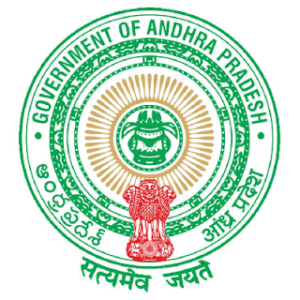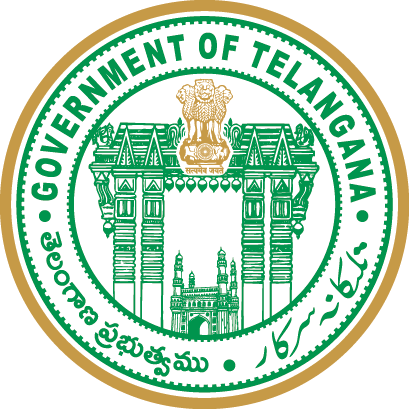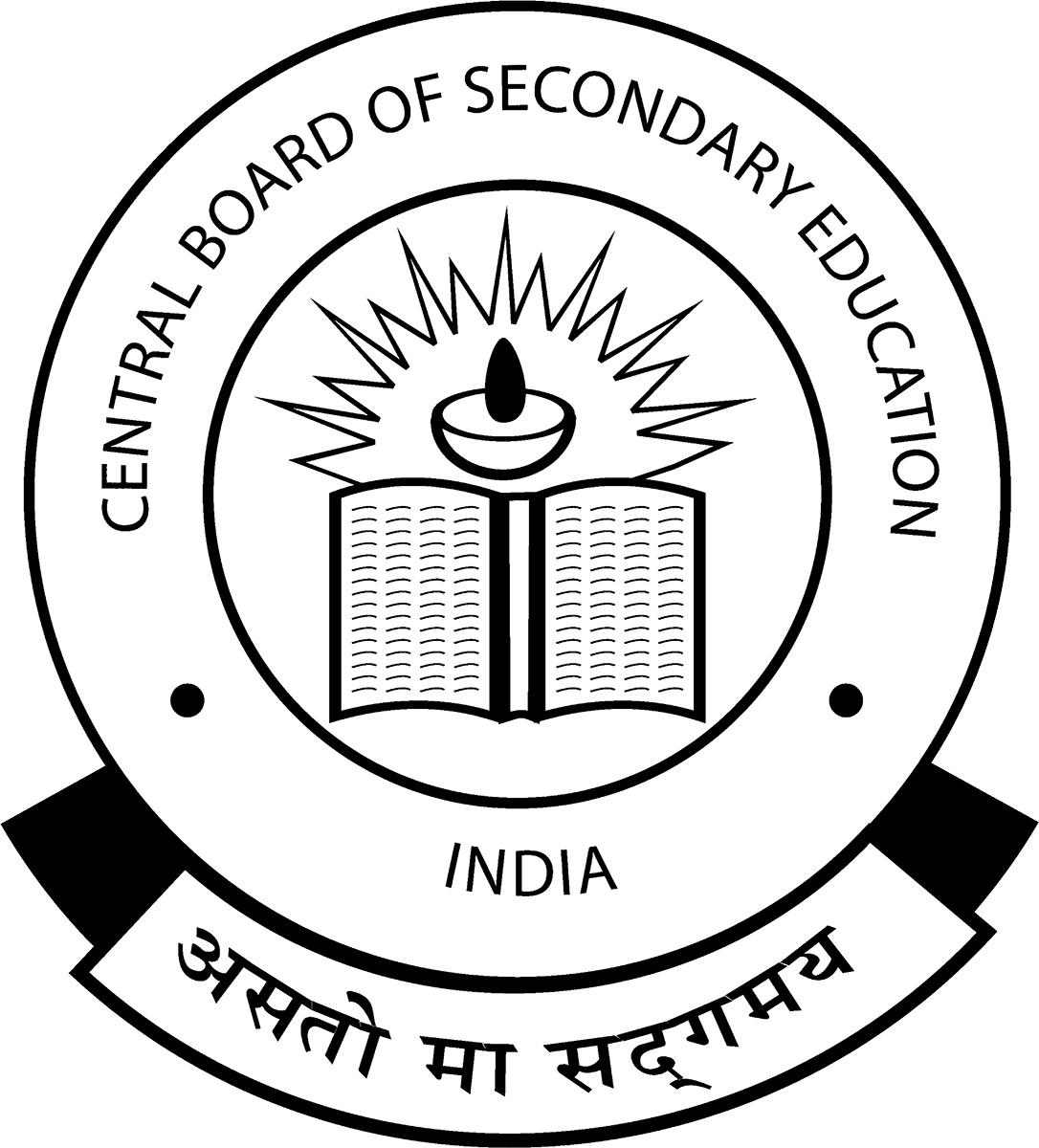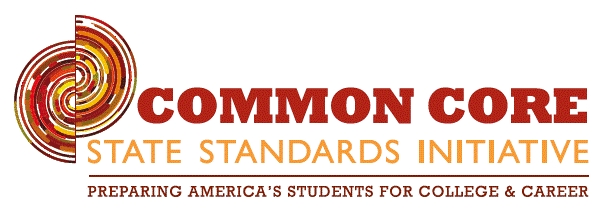## Comprehensive curriculum for your kids

Prepare to test your limits: calculus skills are now available for the below grades:

LKG

#### LKG

Counting up to 5, Counting up to 10, Concept of color, Position and size, Classification,

UKG

#### UKG

Counting up to 20, Comparing, Number names, Geometry shapes, Addition, Subtraction, Sorting ordering, Data Graphs, Measurement, Time,

G-1

Playing with numbers 1, Playing with numbers 2, Skip counting, Ordinal numbers, Addition facts and fun, Subtraction facts and fun, Addition subtraction together, Fractions, Location, Shapes, Time, Dat

G-2

Numbers patterns, Numbers and their Values, Number comparing sequencing, Addition facts and fun, Subtraction facts and fun, Adding and subtracting together, Multiplication, Division, Fractions, Shapes

G-3

Numbers and numeration, Numbers and numeration Introducing thousands, Roman numerals, Addition, Subtraction, Multiplication, Division, Fractions, Geometrical shapes, Measurement, Time, Data handling,

G-4

Number sense, Multiplication, Division, Add, subtract, divide and multiply, Fractions, Factors and multiples, Decimals, Metric measures, Geometry, Probability and statistics, Data and graphs, Patterns

G-5

Number and place values, Multiplication, Division, Decimals, Fractions, Percentage, ratio and proportion, Profit and Loss, Time, Data and graphs, Geometry, Math Explorers, Bigger numbers, Multiply and

G-6

Number system, Factors and multiples, Whole numbers, Integers, Fractions, Simplifications, Decimals, Algebraic expressions, Linear equations in one variable, Ratio, proportion and unitary method, Line

G-7

Integers, Fractions, Decimals, Rational numbers, Exponents and powers, Algebraic expressions, Linear equations in one variable, Ratio and proportion, Unitary method, Percentage, Profit and loss, Simpl

G-8

Rational Numbers, Integers, Linear equations in one variables, Quadrilaterals, Exponents and powers, Comparing quantities using proportions, Square roots and cube roots, Frequency distribution tables

G-9

Real Numbers, Polynomials and Factorisation, The Elements of Geometry, Lines and Angles, Co-Ordinate Geometry, Linear Equations in Two Variables, Triangles, Quadrilaterals, Statistics, Surface Areas a

G-10

Real Numbers, Sets, Polynomials, Pair of Linear Equations in Two Variables, Quadratic Equations, Progressions, Coordinate Geometry, Similar Triangles, Tangents and Secants to a Circle, Mensuration, Tr

GRM

#### GRAMMAR

The sentence, Subject and predicate, Phrase and clause, Nouns, A noun as gender, A noun as number, Pronouns, Adjectives, Modals and auxiliaries, Article, Verb, Adverbs, Adverbs and adjectives, Prepo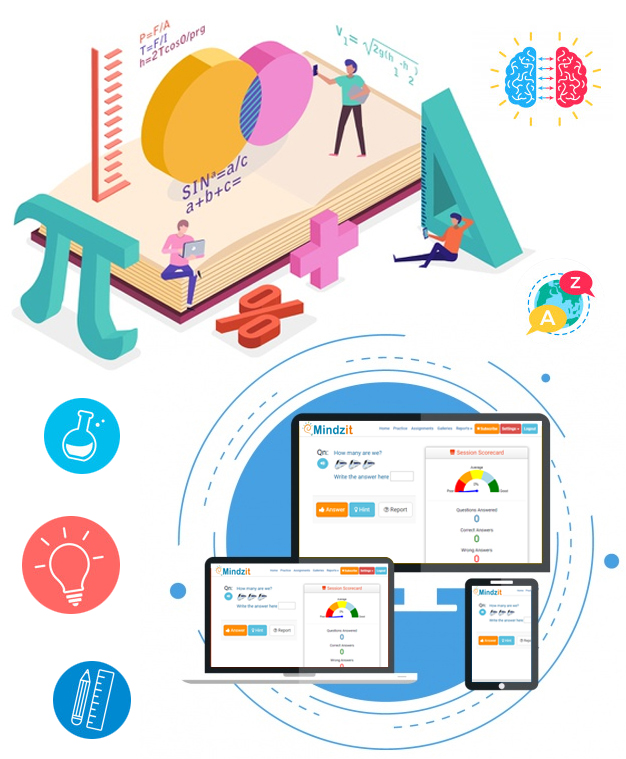### End to End online learning experience between Teacher and Children

Make your students and children unstoppable.

#### Compatible for all devices and renders properly

Explore the benefits by becoming a mindzit member! Your kids will be champions in few days

• All Champions
• Top Performers### 103

Topics- 7 | Questions- 103### 36

Topics- 4 | Questions- 36### 8

Topics- 1 | Questions- 8### 62%

Topics- 1 | Questions- 8### 52%

Topics- 4 | Questions- 36### 44%

Topics- 7 | Questions- 103

## New and Upcoming Features

View the list of new and upcoming robust features to make your kid feel this app more enjoyable.#### Audible questions

• All text based questions are audible#### Topic summary

• A brief summary of the concepts for the given topic for a quick reference#### Select "Don't know" option

• Student can select option "Don't know" which will display the topic synopsis#### New context help

• Option available to every user to know about every context